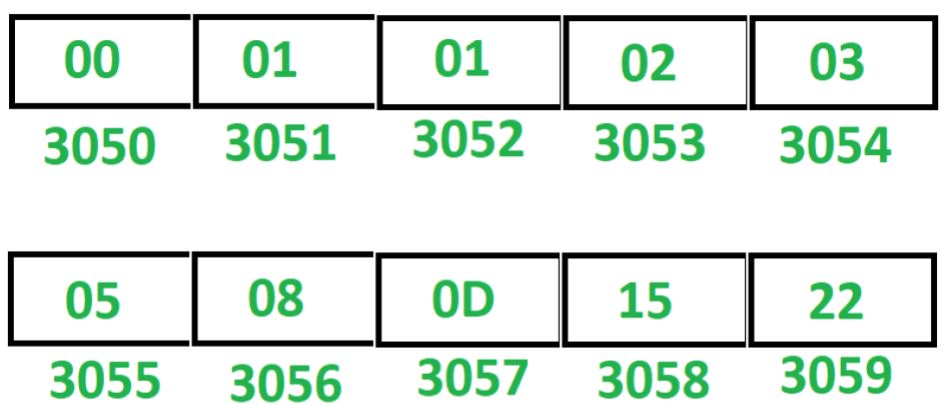# 8085 program to generate Fibonacci series

Problem – Write an assembly language program in 8085 microprocessor to generate Fibonacci series.

Example – Assume Fibonacci series is stored at starting memory location 3050.Note – This program generates Fibonacci series in hexadecimal numbers.

Algorithm –

1. Initialize register H with 30 and register L with 50, so that indirect memory M points to memory location 3050.
2. Initialize register B with 00, register C with 08 and register D with 01.
3. Move the content of B in M.
4. Increment M by 1 so that M points to next memory location.
5. Move the content of D in M.
6. Move the content of B in accumulator A.
7. Add the content of D in A.
8. Move the content of D in B.
9. Move the content of A in D.
10. Increment M by 1 so that M points to next memory location.
11. Move the content of A in M.
12. Decrements C by 1.
13. Jump to memory location 200C if ZF = 0 otherwise Halt the program.

Program –

2000 LXI H, 3050 H <- 30, L <- 50
2003 MVI C, 08 C <- 08
2005 MVI B, 00 B <- 00
2007 MVI D, 01 D <- 01
2009 MOV M, B M <- B
200A INX H M <- M + 01
200B MOV M, D M <- D
200C MOV A, B A <- B
200D ADD D A <- A + D
200E MOV B, D B <- D
200F MOV D, A D <- A
2010 INX H M <- M + 01
2011 MOV M, A M <- A
2012 DCR C C <- C – 01
2013 JNZ 200C Jump if ZF = 0
2016 HLT END

Explanation – Registers A, B, C, D, H, L are used for general purpose.

1. LXI H 3050: assigns 30 to H and 50 to L.
2. MVI B, 00: assigns 00 to B.
3. MVI C, 08: assigns 08 to C.
4. MVI D, 01: assigns 01 to D.
5. MOV M, B: moves the content of B in M.
6. INX H: increment M by 1.
7. MOV M, D: moves the content of D in M.
8. MOV A, B: moves the content of B in A.
9. ADD D: add the content of D and A. Store the result in A.
10. MOV B, D: moves the content of D in B.
11. MOV D, A: moves the content of A in D.
12. INX H: increment M by 1.
13. MOV M, A: moves the content of A in M.
14. DCR C: decrements C by 1.
15. JNZ 200C: jump to memory location 200C if ZF = 0.
16. HLT: stops executing the program and halts any further execution.

Don’t stop now and take your learning to the next level. Learn all the important concepts of Data Structures and Algorithms with the help of the most trusted course: DSA Self Paced. Become industry ready at a student-friendly price.

My Personal Notes arrow_drop_upCheck out this Author's contributed articles.

If you like GeeksforGeeks and would like to contribute, you can also write an article using contribute.geeksforgeeks.org or mail your article to contribute@geeksforgeeks.org. See your article appearing on the GeeksforGeeks main page and help other Geeks.

Please Improve this article if you find anything incorrect by clicking on the "Improve Article" button below.